## LetsPlayMaths.Com

WELCOME TO THE WORLD OF MATHEMATICS

# Class 6 Triangle

Triangle

Equilateral Triangle

Isosceles Triangle

Scalene Triangle

Acute Triangle

Right Triangle

Obtuse Triangle

Isosceles Right-Angle Triangle

Perimeter of a triangle

Properties of Triangle

Angles Test

Angles Worksheet

## Triangle

If P, Q, R are three non-collinear points, the figure made up by three line segments PQ, QR and RP is known as triangle with P, Q and R as vertices.We shall use the symbol △ PQR to denote the triangle. The three line segments PQ, QR and RP are called the sides of the triangle.

The three angles ∠RPQ , ∠PQR, and ∠QRP are known as angles of the triangle. We can denote this angles as ∠P, ∠Q and ∠R respectively.

There are various types of tringles as mentioned below.

1. Equilateral Triangle
2. Isosceles Triangle
3. Scalene Triangle
4. Acute Triangle
5. Right Triangle
6. Zero angle
7. Obtuse Triangle
8. Isosceles Right-Angle Triangle

## Equilateral Triangle

A triangle whose all sides are equal is known as equilateral triangle.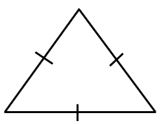## Isosceles Triangle

A triangle whose two sides are equal is known as isosceles triangle.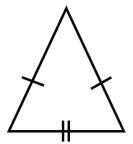## Scalene Triangle

A triangle whose no two sides are equal is known as scalene triangle.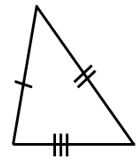## Acute Triangle

A triangle whose each angle is less than 90° is known as acute triangle.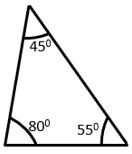## Right Triangle

A triangle whose one angle is equal to 90° is known as right angled triangle or right triangle.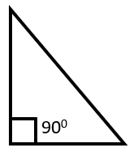## Obtuse Triangle

A triangle whose one angle is more than 90° is known as obtuse triangle.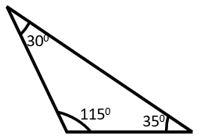## Isosceles Right-Angle Triangle

A triangle whose one angle is equal to 90° and it's two sides are equal then it is known as isosceles right angle triangle.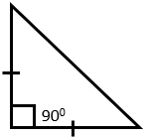## Perimeter of a triangle

The sum of the lengths of the sides of a triangle is known as it's perimeter.

Example 1. Find the perimeter of the below triangle.Solution. PQ = 5cm, PR = 4 cm and QR = 7 cm
Perimeter = PQ + PR + QR
= 5 + 4 + 7 = 16 cm
So, the perimeter of the triangle is 16 cm.

Example 2. Find the perimeter of the below triangle.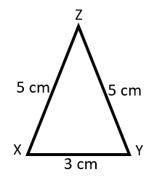Solution. XY = 3cm, YZ = 5 cm and ZX = 5 cm
Perimeter = XY + YZ + ZX
= 3 + 5 + 5 = 13 cm
So, the perimeter of the triangle is 13 cm.

## Properties of Triangle

1. If all the angles in a triangle are equal, then its sides are equal.

2. If all the sides of a triangle are equal, then angles are also equal.

3. If two angles of a triangle are equal, then it has two equal sides.

4. If two sides of a triangle are equal, then its two angles are also equal.

5. If none of the angles of a triangle are equal, then none of the sides are equal.

6. The sum of all the angles of a triangle is equal to 180°.

7. The sum of lengths of any two sides is greater than the length of the third side.

## Class-6 Triangle Test

Triangle Test - 1

Triangle Test - 2

## Class-6 Triangle Worksheet

Triangle Worksheet - 1

Triangle Worksheet - 2

Triangle Worksheet - 3